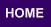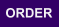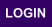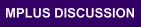Mplus
Thursday
June 08, 2023Mplus Mplus at a GlanceGeneral DescriptionMplus Programs Pricing Version History System Requirements Platforms
 Analyses/ResearchMplus Examples Papers References
 Search

Mplus Base Program
What's New In Version 3

SEM

• New features for categorical outcomes
•  Regression, path analysis, factor analysis, SEM, growth modeling using ML-EM ML-EM using numerical integration ML missing data under MAR WLMSV missing data under covariate-MAR WLSMV chi-square difference testing WLSMV modification indices

• New types of outcomes
•  Counts - Poisson and zero-inflated Poisson modeling Censored - Censored-normal and censored-inflated normal     Regression, path analysis, factor analysis, SEM, growth modeling using ML-EM     ML-EM using numerical integration     ML missing data under MAR

• Random Slopes
•  Categorical outcomes Slopes for exogenous observed variables Slopes for endogenous observed variables Slopes for continuous latent variables

• Interactions between continuous latent variables and between continuous latent variables and observed variables using ML
•  Continuous factor indicators Categorical factor indicators

• Non-linear factor analysis

• Indirect effects

• Bootstrap standard errors and confidence intervals

• Nonlinear constraints
• Growth Modeling

• New language

• Categorical outcomes using ML-EM via numerical integration

• Two-part growth modeling

• Interaction modeling, for example, between initial status and a time-invariant covariate

• Automatic starting values for growth factor means and variances for continuous outcomes